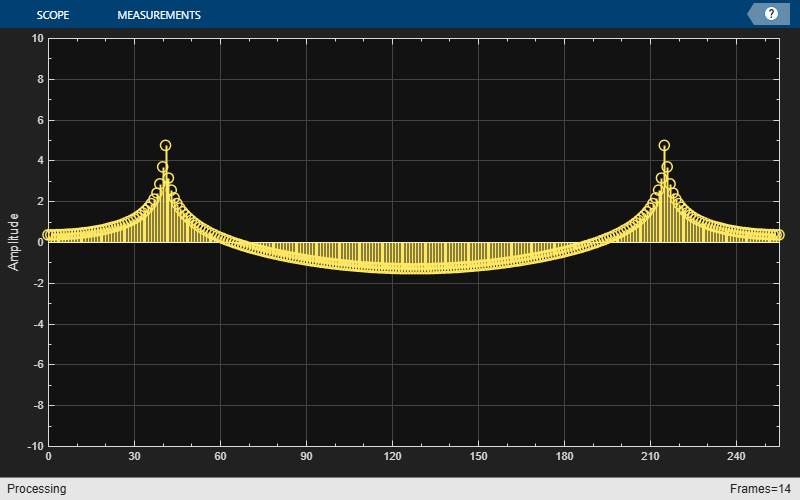Main Content

# write

Write data to buffer

## Syntax

``nOverrun = write(asyncBuff,x)``

## Description

example

````nOverrun = write(asyncBuff,x)` writes the input array, `x`, to the buffering object, `asyncBuff`, and returns the number of samples overrun, `nOverrun`.```

## Examples

collapse all

Write a sine wave of variable frame size to the buffer. Compute the FFT of the sine wave and visualize the result on an array plot.

Initialize the `dsp.AsyncBuffer`, `dsp.ArrayPlot`, and `dsp.FFT` System objects.

```asynBuff = dsp.AsyncBuffer; plotter = dsp.ArrayPlot; fftObj = dsp.FFT('FFTLengthSource','Property','FFTLength',256); ```

The sine wave is generated using the `sin` function in MATLAB. The `start` and `finish` variables mark the start and finish indices of each frame. If enough data is cached, read from the buffer and perform the FFT. View the FFT on an array plot.

```start = 1; for Iter = 1 : 2000 numToWrite = randi([200,800]); finish = start + numToWrite; inputData = sin(start:finish)'; start = finish + 1; write(asynBuff,inputData); while asynBuff.NumUnreadSamples >= 256 x = read(asynBuff,256); X = abs(fftObj(x)); plotter(log(X)); end end ```## Input Arguments

collapse all

Async buffer, specified as a `dsp.AsyncBuffer` System object.

Data written to the buffer, specified as a vector or a matrix. The maximum number of rows in the buffer is determined by the `Capacity` property of `asyncBuff`. The number of channels in the buffer is determined by the second dimension of the first data written to the buffer. Successive data inputs can vary in the number of rows, but the number of channels must remain fixed. To change the number of channels, you must call `release` on the buffer.

For example, the following is accepted:

```asyncBuff = dsp.AsyncBuffer; % First call to write write(asyncBuff,randn(15,5)); % Add more data with a different number of rows write(asyncBuff,randn(25,5)); write(asyncBuff,randn(5,5));```

The following is not accepted and errors out:

```asyncBuff = dsp.AsyncBuffer; % First call to write write(asyncBuff,randn(15,5)); % Add more data with a different number of columns write(asyncBuff,randn(15,15));```

To change the number of channels, call `release` on the buffer.

```asyncBuff = dsp.AsyncBuffer; % First call to write write(asyncBuff,randn(15,5)); release(asyncBuff) % Add more data with a different number of columns write(asyncBuff,randn(15,15));```

Data Types: `single` | `double` | `int8` | `int16` | `int32` | `int64` | `uint8` | `uint16` | `uint32` | `uint64` | `logical`
Complex Number Support: Yes

## Output Arguments

collapse all

Number of samples overrun in the current call to `write`. The number of samples overrun is the number of unread samples overwritten. If `x` is a multichannel input, then `nOverrun` is the number of rows of data overrun.

Data Types: `int32`

## See Also

### Objects

Introduced in R2017a

Watch now